## Example Questions

← Previous 1

### Example Question #1 : How To Find The Square Root Of A Decimal

Find the square root of the following decimal: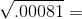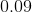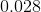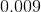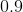Explanation:

The easiest way to find the square root of a fraction is to convert it into scientific notation.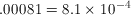The key is that the exponent in scientific notation has to be even for a square root because the square root of an exponent is diving it by two. The square root of 9 is 3, so the square root of 8.1 is a little bit less than 3, around 2.8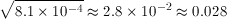### Example Question #1 : How To Find The Square Root Of A Decimal

Find the square root of the following decimal: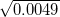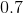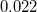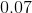Explanation:

To find the square root of this decimal we convert it into scientific notation.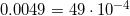Because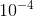has an even exponent, we can divide the exponenet by 2 to get its square root.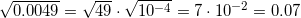### Example Question #1 : How To Find The Square Root Of A Decimal

Find the square root of the following decimal: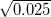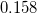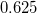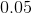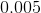Explanation:

This problem can be solve more easily by rewriting the decimal into scientific notation.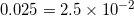Because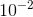has an even exponent, we can take the square root of it by dividing it by 2. The square root of 4 is 2, and the square root of 1 is 1, so the square root of 2.5 is less than 2 and greater than 1.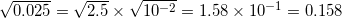### Example Question #2 : How To Find The Square Root Of A Decimal

Find the square root of the following decimal: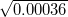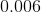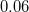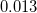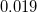Explanation:

This problem becomes much simpler if we rewrite the decimal in scientific notation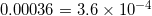Becausehas an even exponent, we can take its square root by dividing it by two. The square root of 4 is 2, and because 3.6 is a little smaller than 4, its square root is a little smaller than 2, around 1.9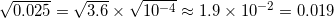### Example Question #3 : How To Find The Square Root Of A Decimal

Find the square root of the following decimal: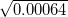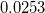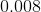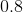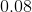Explanation:

To find the square root of this decimal we convert it into scientific notation.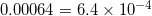Becausehas an even exponent, we can divide the exponenet by 2 to get its square root. The square root of 9 is 3, and the square root of 4 is two, so the square root of 6.4 is between 3 and 2, around 2.53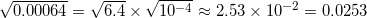### Example Question #22 : Decimals

Find the square root of the following decimal: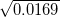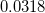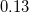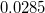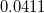Explanation:

To find the square root of this decimal we convert it into scientific notation.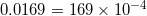Becausehas an even exponent, we can divide the exponenet by 2 to get its square root.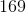is a perfect square, whose square root is.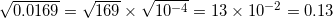### Example Question #1 : How To Find The Square Root Of A Decimal

Find the square root of the following decimal: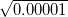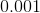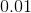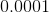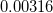Explanation:

To find the square root of this decimal we convert it into scientific notation.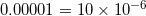Because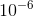has an even exponent, we can divide the exponenet by 2 to get its square root. The square root of 9 is 3, so the square root of 10 should be a little larger than 3, around 3.16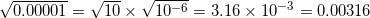### Example Question #1 : How To Find The Square Root Of A Decimal

Find the square root of the following decimal: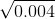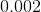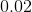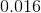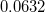Explanation:

To find the square root of this decimal we convert it into scientific notation.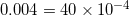Becausehas an even exponent, we can divide the exponenet by 2 to get its square root. The square root of 36 is 6, so the square root of 40 should be a little more than 6, around 6.32.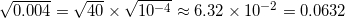### Example Question #11 : Basic Squaring / Square Roots

Find the square root of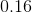.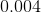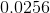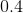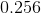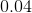Explanation:

Rewrite the expression in radical form.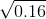Rewrite the decimal with factors and simplify.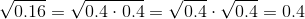### Example Question #1 : How To Find The Square Root Of A Decimal

Find the square root of.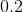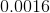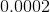Explanation:

Rewrite the question in radical form.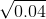Split upinto its common factor.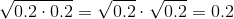← Previous 1